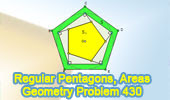## Sunday, March 14, 2010

### Problem 430: Circumscribed and Inscribed Regular Pentagon, Perpendicular, Areas

Proposed Problem
Click the figure below to see the complete problem 430 about Circumscribed and Inscribed Regular Pentagon, Perpendicular, Areas.Complete Problem 430

Level: High School, SAT Prep, College geometry

1.S/7=OAB-OA'B', S1/7=OA''B''.
OAB:OA'B':OA''B''=AB^2:A'B'^2:A''B''^2
AB^2-A'B'^2=A''B''2
Then, S/7=S1/7
q.e.d.

2.To anonymous:
7 or 5?

3.name h altitude of A'B'C'D'E'
from similarity of AOE and A'OE'
a/R = b/h =>

R = ah/b (1)

join E' to B' and name G E'B' meet A"B" we get
right triangle E'A'G with A'E' = b, A'G = c , GE' =a)
( B'B"G is isoceles B'B" = B"G = ...A'A" )

from similarity of A'OM (M midpoint of A'E')
and E'A'G ( right tr and angle 36°)
(b/2)/R = c/a
substitute R from (1)

h = b²/2c (2)
=>
R = ab/2c (3)

from similarity of A"ON ( N midpoint of A"E") and GA'E' (c/2)/h1 = c/b ( h1 altitude of pentagon C)

h1 = b/2 (4)

Sa = (5∙a ∙R)/2

Sa = 5a²b/4c ( R from 3)

Sb = ( 5∙b∙h )/2

Sb = 5b³/4c ( h from 2)
=>
Sj = 5a²b/4c - 5b³/4c = 5b(a² - b²)/4c ( a²-b²=c²)

Sj = 5bc/4 (5)

Sc = 5∙c∙h1/2

Sc = 5bc/4 (6) ( h1 from 4)

comparing (5) and (6) give the result

4.the area of a regular pentagon with side s is:
A=s²(5/4)cotan36
and we know (previous problem) that c²=a²-b²....
.-.

5.Masterstream said…

The sweetest proof seems to be this:-

Let a,b,c be the side-lengths of pentagons ABCDE, A'B'C'D'E', A''B''C''D''E'' respectively, as in Problem 429(q.v.); and let S2,S3 be the areas of the inscribed regular pentagon A'B'C'D'E' and the circumscribed regular pentagon ABCDE respectively.

Since all regular pentagons are similar,
S3:S2:S1 = a^2:b^2:c^2.

So S:S1 = (S3–S2):S1 = (a^2–b^2):c^2 = 1 ,
(since a^2–b^2 = c2, by Problem 429 [q.v.])

i.e. S = S1 . QED